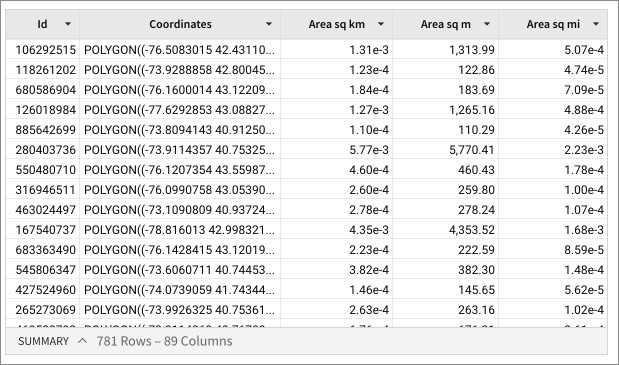# Area

The Area function calculates the area of a geography polygon, in specified units.

When you apply the Area function to geography points or geography lines, Sigma returns 0.

You can use the Area function on Snowflake and BigQuery connections.

Area is one of Sigma's Geography functions.

## Syntax

`Area(units, polygon)`

The function arguments are:

units
Required
The unit of measurement for the area
Valid values are `"square_kilometers"`, `"square_meters"`, and `"square_miles"`.
polygon
Required
The object for which we calculate the area
Must be in valid Geography polygon format.
To work from known latitude and longitude values, use the MakePoint or MakeLine functions.

## Examples

`Area("square_kilometers", [Coordinates])Area("square_meters", [Coordinates])Area("square_miles", [Coordinates])`

The Area function returns the following values for the Coordinates column values that define a polygon, when specifying area in square kilometers, square meters, or square miles: